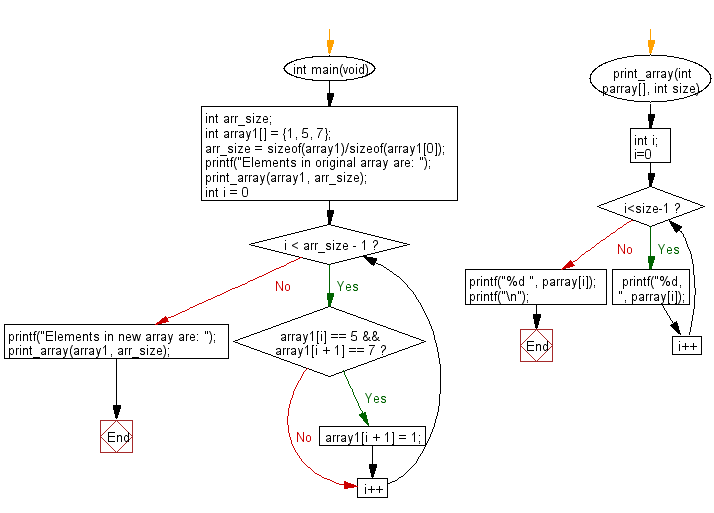﻿ C Program: Create an array of 3 integers from an array# C Exercises: Check a given array of integers of length 3 and create a new array

## C-programming basic algorithm: Exercise-44 with Solution

Write a C program to check a given array of integers of length 3 and create a new array. If there is a 5 in the given array immediately followed by a 7 then set 7 to 1.

C Code:

``````#include <stdio.h>
#include <stdlib.h>
int main(void){
int arr_size;
int array1[] = {1, 5, 7};
arr_size = sizeof(array1)/sizeof(array1);
printf("Elements in original array are: ");
print_array(array1, arr_size);
for (int i = 0; i < arr_size - 1; i++)
{
if (array1[i] == 5 && array1[i + 1] == 7)
array1[i + 1] = 1;
}
printf("Elements in new array are: ");
print_array(array1, arr_size);
}
print_array(int parray[], int size)
{
int i;
for( i=0; i<size-1; i++)
{
printf("%d, ", parray[i]);
}
printf("%d ", parray[i]);
printf("\n");
}
``````

Sample Output:

```Elements in original array are: 1, 5, 7
Elements in new array are: 1, 5, 1
```

Pictorial Presentation:Flowchart:C Programming Code Editor:

What is the difficulty level of this exercise?

Test your Programming skills with w3resource's quiz.

﻿

## C Programming: Tips of the Day

Why doesn't a+++++b work?

printf("%d",a+++++b); is interpreted as (a++)++ + b according to the Maximal Munch Rule!.

++ (postfix) doesn't evaluate to an lvalue but it requires its operand to be an lvalue.

! 6.4/4 says the next preprocessing token is the longest sequence of characters that could constitute a preprocessing token"

Ref : https://bit.ly/3fdldUT